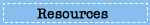# Sample Questions

## What questions are used in the NAEP mathematics assessment?

Explore sample questions from the mathematics assessment, and see how the NAEP mathematics questions relate to student performance.## Test Yourself in Mathematics

Select one of the grade tabs below to try out questions from the 2011 NAEP mathematics assessment. After answering all the questions, explore your results and question commentaries.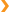Grade 4 Sample Questions

# Question 1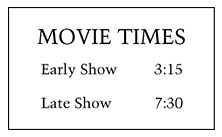The early show and the late show for a movie last the same amount of time. The early show begins at 3:15 P.M. and ends at 4:27 P.M. The late show begins at 7:30 P.M. At what time does the late show end? Enter your answer in the box below.

# Question 2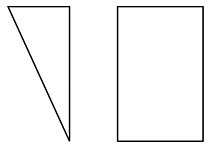How are the right triangle and the rectangle alike?

A. Each figure has at least one right angle.
B. Each figure has parallel sides.
C. Each figure has at least one line of symmetry.
D. Each figure has at least two sides that are the same length.

# Question 3

Subtract: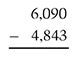A. 1,147
B. 1,247
C. 2,257
D. 2,853

# Question 4

Sam folds a piece of paper in half once. There are 2 sections.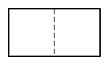Sam folds the paper in half again. There are 4 sections.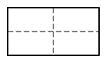Sam folds the paper in half again. There are 8 sections.

Sam folds the paper in half two more times.

Which list shows the number of sections there are each time Sam folds the paper?

A. 2, 4, 8, 10, 12
B. 2, 4, 8, 12, 24
C. 2, 4, 8, 16, 24
D. 2, 4, 8, 16, 32

# Question 5

What number is 10,000 more than 333,333?

A. 333,433
B. 334,333
C. 343,333
D. 433,333

# Question 6

The graph below shows students’ favorite fruits.

Use these clues to label the bars with the correct fruit.

• Twice as many students chose apples as grapes.
• Five more students chose peaches than apples.
• Ten more students chose bananas than peaches.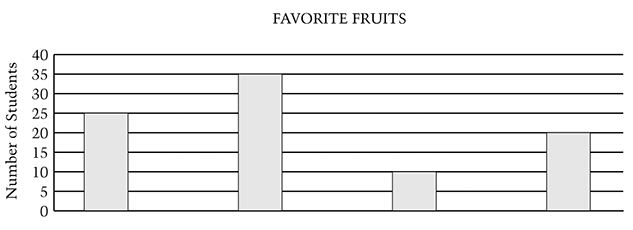Write the correct fruit in the boxes above.

# Question 7

Park School has 316 students. For field day, the students are put into 4 teams with the same number of students on each team. How many students are on each team?

A. 79
B. 312
C. 320
D. 1,264

•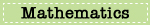•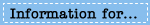•